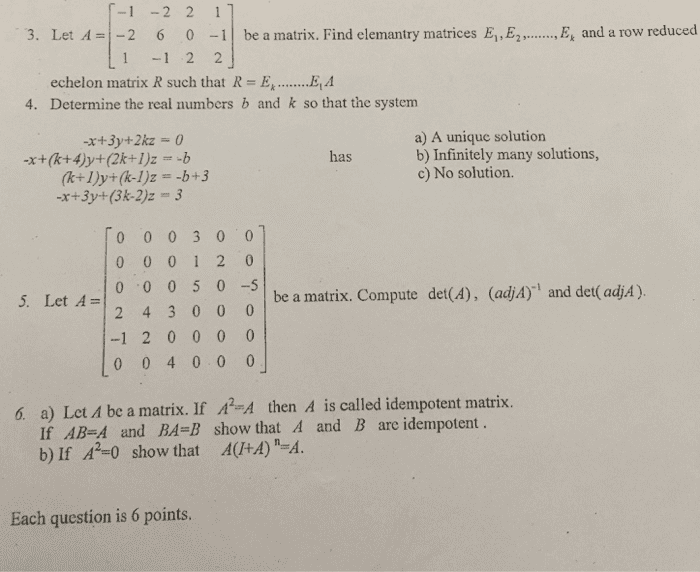1
0
watching
97
views10 Nov 2019

#3. LetA 1-2 L1 -1 2 2 6 0-1| be a matrix. Find elemantry matrices Ei,E2, , E, and a row reduced echelon matrix R such that R 4. Determine the real numbers b and k so that the system a) A unique solution b) Infinitely many solutions, c) No solution. has -x+3y+(3k-2)z 3 0003 0 0 5. Let 4- 0 0050 2 4 3 00 0 be a matrix. Compute det(A), (adjA) and det(CadjA ) -120000 0 0 4 000 a) Let Ð be a matrix. If A2,-A If AB-A and BA-B show that A and B are idempotent b) If A2-0 show that A(+A) -A. 6. then A is called idempotent matrix. Each question is 6 points.

For unlimited access to Homework Help, a Homework+ subscription is required.Sixta KovacekLv2
9 Oct 2019

## Unlock all answers

Get 1 free homework help answer.